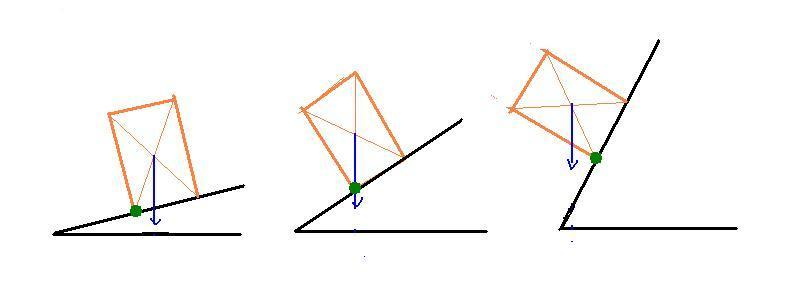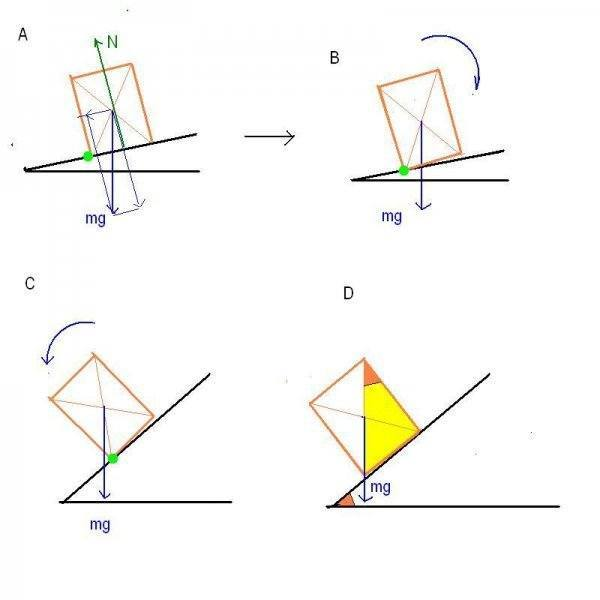# How do I calculate the angle of rotation?

## Homework Statement

We consider Rectangle Parallelepiped on a board and a=1m ; b=2m and c=3m.

The face a and b is considered touching the board.

there’s no sliding; we tilt the board so a is horizontal.

Q: Starting from which angle we can see a rotation?

## Homework Equations

I want to know how am I supposed to know how to find the angle precisely!

## The Attempt at a Solution

Sorry I'm not native English speaker, I translated my thing to here. I suck at Physics so I don't really even know where to begin with. I tried so many things, I've been staring at this for hours. It was supposed to be a chapter about moment of force but maybe I missed something the only formula I have about it is moment of force=Force * Distance :/ Help!

#### Attachments

•Capture d’écran 2015-03-30 à 03.08.50.png
2.3 KB · Views: 424

ehild
Homework Helper
Hi Rehzhor, welcome to PF.

The rules of the Forum require that you show your attempt to solve the problem. If you tried so many things, show at least one of them.
Try some experiment, it will give you ideas. A matchbox on a hard cover of a book, for example.
Look at the picture, when does the box turn over the edge shown in green? (The blue arrow represents the force of gravity.)I wrote them on my board, so I wiped them when I figured out they were wrong. :/

As for the match box I'll say it's in the second and third picture? I mean the center of the gravity looks only good on the first one. (Thanks for helping me out! I really suck at this.)

andrevdh
Homework Helper
It seems they want to know at what angle, when you lift it up, will the structure begin to topple or fall over.
Why would it want to topple over?
What force would or could bring this about?
What changes at this specific angle so that it actually falls over?
As ehild suggests try some experiments. Use plastic bottles or empty soda cans or other objects.

ehild
Homework Helper
I wrote them on my board, so I wiped them when I figured out they were wrong. :/

As for the match box I'll say it's in the second and third picture? I mean the center of the gravity looks only good on the first one. (Thanks for helping me out! I really suck at this.)
Yes, the second picture is the limiting case. You increase the angle of the board just a little, the box will topple over, like In the third case.
Look at the torque of gravity. In what direction does it "try" to turn the box in the pictures? When can it turn the box ?
What is the torque in the second picture? What is the angle the board makes with the horizontal in that case?

Hello everyone, thank you all for your reply and sorry I was at school. I will read all the things you guys wrote and try to understand it. I'll do my best. :D

It seems they want to know at what angle, when you lift it up, will the structure begin to topple or fall over.
Why would it want to topple over?
What force would or could bring this about?
What changes at this specific angle so that it actually fallsAs ehild suggests try some experiments. Use plastic bottles or empty soda cans or other objects.

A: The mass would want to topple over because the center of gravity moved too much to one side due to the angle.
A: Newton?
A: I am not really sure how to explain this one, but I think I got the idea. So let's say I tilt my pen to the left, the gravity P will apply 100% on point A (the lowest point of the pen) and on point B it will only apply some of the mass depending on the angle. I remember I did that once on an exercice but I can't quite remember it well.

#### Attachments

Yes, the second picture is the limiting case. You increase the angle of the board just a little, the box will topple over, like In the third case.
Look at the torque of gravity. In what direction does it "try" to turn the box in the pictures? When can it turn the box ?
What is the torque in the second picture? What is the angle the board makes with the horizontal in that case?

(I am not sure what the torque of gravity is)
A: In the pictures, the box are trying to turn to the left?
A: It turns the box when the box is not touching the book anymore.
A: I am not sure how I can answer that. I think it's starting here that it's bugging me, I have no idea how we do the angles. :/

ehild
Homework Helper
(I am not sure what the torque of gravity is)
Do you know what is the torque of a force with respect to an axis?

andrevdh
Homework Helper
The centre of gravity is a fixed point with respect to the body and do not change its position
as the body is rotated or moved about, that is it moves with the body. It is the point where
the resultant of the weight of the body acts, in this case at the midpoint of the structure. In order
for the structure to stay upright the weight needs to be cancelled by another force acting on the
body in the opposite direction. Where would such a force come from?

Do you know what is the torque of a force with respect to an axis?

Yes I think I do. It's distance * force right?

The centre of gravity is a fixed point with respect to the body and do not change its position
as the body is rotated or moved about, that is it moves with the body. It is the point where
the resultant of the weight of the body acts, in this case at the midpoint of the structure. In order
for the structure to stay upright the weight needs to be cancelled by another force acting on the
body in the opposite direction. Where would such a force come from?

Oh, I've studied something that I call Barycentre and I thought the translation of it was center of gravity, I guess I was wrong haha. I think it's canceled by the a force we call resistance? Like when there's a force applying there's always a reaction coming from the support, right?

Also I have tried to do the thing myself with the help of a friend who's not really sure of how to do neither, we got helped by a third guy but we're still unsure if we were allowed to do what he did.
So here's what he told us to do: http://imgur.com/LiXXoTF

And I used different color as we were progressing, so here:

1) Told me to identify the force (red)
2) Create another angle we'll call it Beta
3) Find Beta.
4) Conclude that the sinus of the angle is equal to beta thus equals to alpha.

Clarification:

Red: Vector P is the gravity ; Vector T is the reaction contrary to the gravity so -vector P
Blue: Created some points and the angle Beta; so here:
=>The surface of the solid is b*a
=>Let's say beta is B'OC' (Rectangle in B')
=>O is the median of c*b
=>C' is in the intersection of c and b and it touches the board.
=>B' is 1/2 of b, also touches the board.
but now we don't have the angle B'OC' so I had to calculate it but I'm missing a measure (C'O) to use pythagore, so here..
Brown: Trying to find C'O
Orange: Finding angle Beta and conclude that it's equal to Alpha

Edit: I mean the whole thing looks a little bit off to me, why did we do all the maths; I didn't feel like I found the right answer by doing what he told me. :/ Kinda missed the "Eureka!" moment of finally understanding.

Last edited:
ehild
Homework Helper
I do not see what you do. Use side view instead of the "3D" picture, as I did in Post #2.

The problem states that there is no sliding, so the net force on the block is zero. You have to consider the torques.
In picture A, you see that the parallel component of mg would rotate the block anti-clockwise, and the torque of the normal component cancels with the normal force N. The force of friction has no torque. If the parallel component of gravity turns the block just a tiny bit away from the slope, the normal force does not act any more: The torque is from gravity alone. In B, gravity would turn the block clockwise around the fulcrum (green ), so it goes back to the initial position, its base touching the slope again. The block is in stable equilibrium.
In case C, gravity would turn the block anti-clockwise around the fulcrum, the block is unstable.
In Case D, the line of attack of gravity goes through the fulcrum. What is its torque? . What happens if you increase a bit the tilt of the the slope?Last edited:
Omg I finally understood, that's what I'm talking about. Thank you sooo much. I'll do it now. I LOVE YOU!